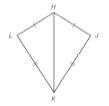Chapter 4.2, Problem 23EElementary Geometry For College St...

7th Edition
Alexander + 2 others
ISBN: 9781337614085

Solutions

Chapter
SectionElementary Geometry For College St...

7th Edition
Alexander + 2 others
ISBN: 9781337614085
Textbook Problem

In Exercise 19 to 24 , complete each proof. Given: Kite H J K L with diagonal H K ¯ Prove: H K ¯ bisects ∠ L H JTo determine

To prove:

HK¯ bisects LHJ

Explanation
 Given: Kite HJKL with diagonal HK¯ Prove: HK¯ bisects ∠LHJ

Calculation:

1) Pair of adjacent sides of a kite are .

Here, pair of adjacent sides are HL¯HJ¯, and LK¯JK¯

2) Identity: H

Still sussing out bartleby?

Check out a sample textbook solution.

See a sample solution

The Solution to Your Study Problems

Bartleby provides explanations to thousands of textbook problems written by our experts, many with advanced degrees!

Get Started

Find the point of intersection of the two straight lines having the equations y =34x + 6 and 3x 2y + 3 = 0.

Applied Calculus for the Managerial, Life, and Social Sciences: A Brief Approach

State Keplers Laws.

Multivariable Calculus

Convert the expressions in Exercises 6584 to power form. 23(x2+1)33(x2+1)734

Finite Mathematics and Applied Calculus (MindTap Course List)

True or False: is monotonic.

Study Guide for Stewart's Single Variable Calculus: Early Transcendentals, 8th

It does not exist.

Study Guide for Stewart's Multivariable Calculus, 8th